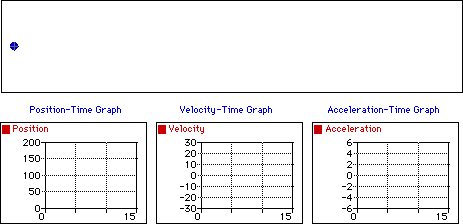## Constant Positive Velocity

Observe that the object below moves with a constant velocity in the positive direction. The dot diagram shows that each consecutive dot is the same distance apart (i.e., a constant velocity). The position-time graph shows that the slope is both constant (meaning a constant velocity) and positive (meaning a positive velocity). The velocity-time graph shows a horizontal line with zero slope (meaning that there is zero acceleration); the line is located in the positive region of the graph (corresponding to a positive velocity). The acceleration-time graph shows a horizontal line at the zero mark (meaning zero acceleration).For more information on physical descriptions of motion, visit The Physics Classroom Tutorial. Detailed information is available there on the following topics:

Ticker Tape Diagrams

Position-Time Graphs

Velocity-Time Graphs